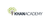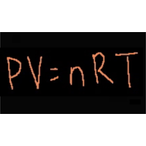# Chemistry: Ideal gas equation example 1

Product type
Total time

## Chemistry: Ideal gas equation example 1Khan Academy

Need more information? Get more details on the site of the provider.### Description

Figuring out the number of moles of gas we have using the ideal gas equation: PV=nRT.

## Chemistry

Did you know that everything is made out of chemicals? Chemistry is the study of matter: its composition, properties, and reactivity. This material roughly covers a first-year high school or college course, and a good understanding of algebra is helpful.

## Ideal gas equation

In this tutorial, Sal shows you how to use the ideal gas equation in calculations.

## Topic: Science

Learn about all the sciences, from physics, chemistry and biology, to cosmology and astronomy, across hundreds of videos, articles and practice questions.

There are no frequently asked questions yet. If you have any more questions or need help, contact our customer service.

Didn't find what you were looking for? See also: Oil & Gas, Chemistry, General Management, Software / System Engineering, and Health Management.

Figuring out the number of moles of gas we have using the ideal gas equation: PV=nRT.

## Chemistry

Did you know that everything is made out of chemicals? Chemistry is the study of matter: its composition, properties, and reactivity. This material roughly covers a first-year high school or college course, and a good understanding of algebra is helpful.

## Ideal gas equation

In this tutorial, Sal shows you how to use the ideal gas equation in calculations.

## Topic: Science

Learn about all the sciences, from physics, chemistry and biology, to cosmology and astronomy, across hundreds of videos, articles and practice questions.

There are no reviews yet.